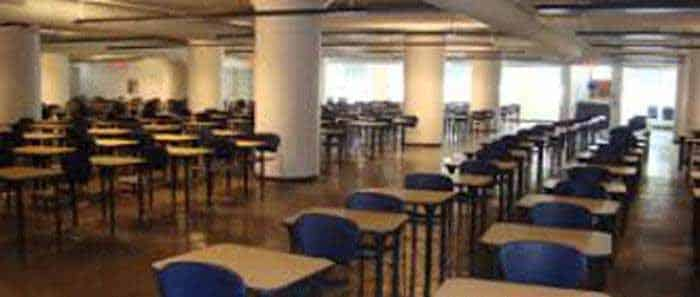Bank sample Question Papers

Seating Arrangement Questions for Bank POQuestion: Six persons A, B, C, D, E and F are sitting in a circle. B is between F and C; A is between E and D; F is to the left of D. Who is between A and F?
(a) B
(b) C
(c) D
(d) A
Ans: (c)

Directions: (next 2 questions): 6 men R, S, T, U, V and W sat around a circular table playing cards. It was noticed that no two men .the initial letters of whose names are adjacent in the alphabetical order, sat next to each other, U was opposite of R. V was not to the immediate right of R.

Question: Who sat to the immediate left of R ?
(a) S
(b) T
(c) V
(d) W
Ans: (c)

Question: Who sat to the immediate right of R ?
(a) S
(b) T
(c) V
(d) W
Ans: (b)

Related: What is 1A coach in Train?

Directions : (next 3 questions): Five persons are sitting in a row. One of the two persons at the extreme ends is intelligent and other one is fair. A fat person is sitting to the right of a weak person. A tall person is to the left of the fair person and the weak person is sitting between the intelligent and the fat person.

Question. Tall person is at which place counting from right ?
(a) First
(b) Second
(c) Third
(d) Fourth
Ans: (b)

Question: Person to the left of weak person possesses which of the following characteristics ?
(a) Intelligent
(b) Fat
(c) Fair
(d) Tall
Ans: (a)

Question: Which of the following persons is sitting at the centre ?
(a) Intelligent
(b) Fat
(c) Fair
(d) Weak
Ans: (b)

Question: Who among P, Q R, S and T is in the middle while standing in a line ?
I. Q is to the right of T
II. S is between P and T
II I. Q is between T and R
(a) P
(b) Q
(c) R
(d) T
Ans: (d)

Related: Sports Trophy names

Directions: (next 4 questions) Read the following information carefully and answer the questions given below it.
I. Eight persons E, F, G, H, l, J, K and L are seated around a square table- two on each side.
II. There are three lady members and they are not seated next to each other.
III. J is between L and F.
IV. G is between I and F.
V. H, a lady member, is second to the left of J.
VI. L, a male member, is seated opposite of E, a lady member,
VII. There is a lady member between F and l.

Question: Who among the following is seated between E and H ?
(a) F
(b) I
(c) J
(d) None of these
Ans: (d)

Question: How many persons are seated between K and F ?
(a) One
(b) Two
(c) Three
(d) Cannot be determined
Ans: (c)

Question: Who among the following are the three lady members?
(a) E, G and J
(b) E, H and G
(c) G, H and J
(d) Cannot be determined
Ans: (b)

Question: Who among the following is to the immediate left of F?
(a) G
(b) I
(c) J
(d) Cannot be determined
Ans: (c)

Question: Which of the following is true about J?
(a) J is a male member
(b) J is a female member
(c) Sex of J cannot be determined
(d) Position of J cannot be determined
Ans: (a)

Directions: (next 4 questions) Study the following information to answer the given questions.
(i) Eight friends A, B, C, D, E, F, G and H are seated in a circle facing centre.
(ii) D is between B and G and F is between A and H.
(iii) E is second to the right of A.

Question: Which of the following is A’s position ?
(a) left of F
(b) Right of F
(c) Between E and F
(d) can’t be determined

Question: Which of the following is C’s position ?
(a) Between E and A
(b) Between G and E
(c) Second to the left of B
(d) Can’t be determined
Ans: (a)

Question: Who are the neighbours of D ?
(a) B and C
(b) C and E
(c) B and G
(d) B and G or B and H
Ans: (c)

Related: Important days of April

Question: If the positions of B and G and D and A are interchanged then who is sitting between B and G in new position.
(a) D
(b) A
(c) H
(d) E
Ans: (b)

Question: If B is sitting opposite to C and H is sitting opposite to E then find who is sitting opposite to F ?
(a) B
(b) G
(c) A
(d) D
Ans: (b)

Question: Four girls are sitting on a bench to be photographed. Shikha is to the left of Reena. Manju is to the right of Reena. Rita is between Reena and Manju. Who would be the second from the left in the photograph ?
(a) Reena
(b) Shikha
(c) Manju
(d) Rita
Ans: (d)